# College Chemistry : Balancing Chemical Equations

## Example Questions

### Example Question #1 : Balancing Chemical Equations

When balanced, what is the value ofin the following chemical equation?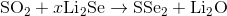Explanation:

Recall that a balanced chemical equation has the same number of each element on one side.Start by counting the number of each element on each side.

There are the following numbers of moles of each reactant: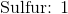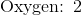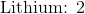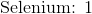There are the following numbers of moles of each product: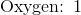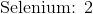Add coefficients in front of the molecular compounds in the equation until there are the same numbers of sulfur, oxygen, lithium, and selenium on each side.

The balanced chemical equation is the following: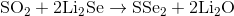Both products and reactants now have the following number of moles: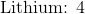Since the coefficient in front of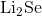ismust equal to.

### Example Question #2 : Balancing Chemical Equations

Balance the following chemical equation: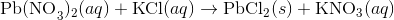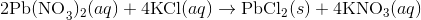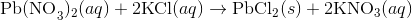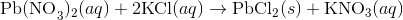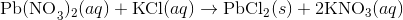Explanation:

A balanced chemical equation will have the same number of each atom on both sides of the reaction.Start by balancing the number of nitrate.

Since we havenitrate on the left, we must also havenitrate on the right.This equation now givespotassium on the left, andpotassium on the right. Balance the potassium.This equation is balanced because there are equal numbers of each atom on both sides.